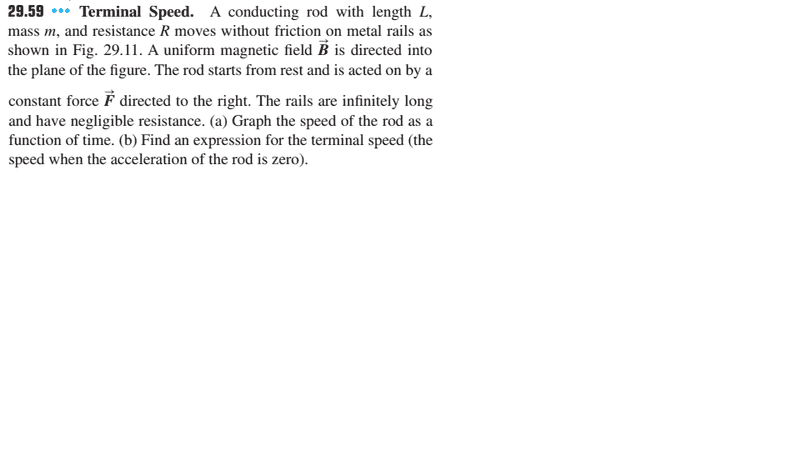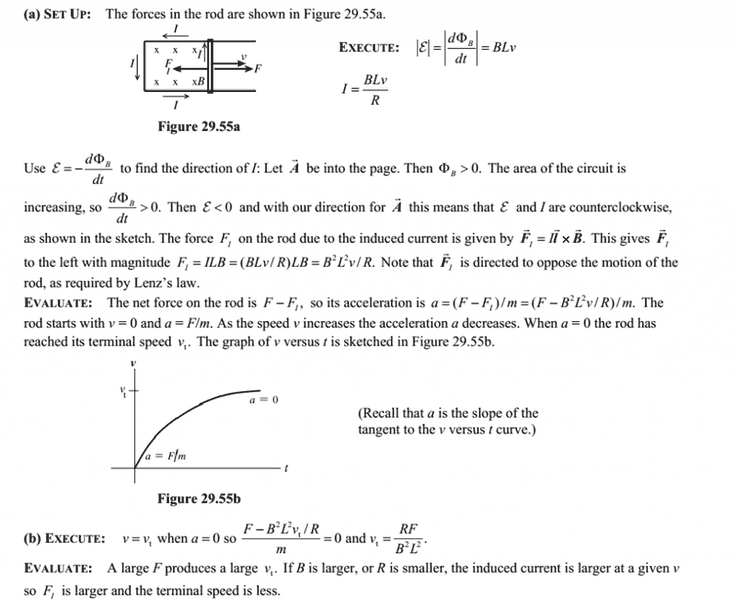# Terminal Speed expression

## Homework Statement## Homework Equations

That is the solution:## The Attempt at a Solution

I think ε≠Bvl because ε = - dø/dt=- Bd(v.t)/dt, v is not constant.
Why ε=Bvl???

## Answers and Replies

CAF123
Gold Member
The induced emf in the loop is related to the change in flux through a surface whose boundary is the loop: $$\epsilon = -\frac{\text{d} \phi}{\text{d}t} = -\frac{\text{d}}{\text{d}t} \int_S \mathbf{B} \cdot \mathbf{dS}$$
In your case, the B field is of constant magnitude and direction and dS instantaneously is Ldx.

•1 person
dø/dt = d(B. Area)/dt = d(BLx)/dt = BL dx/dt = BLv.

•1 person